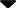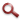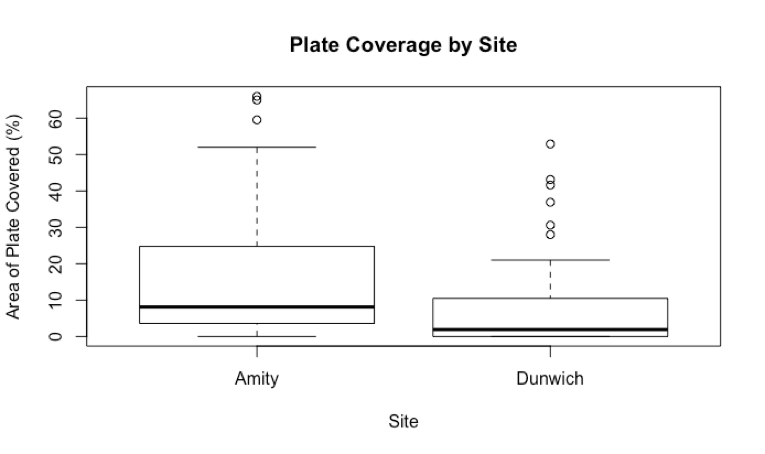Site WebYou are here:

## Student Project FiguresFigure 11 Figure 11: Comparative boxplots of the percentage area of ARMS plate covered by Didemnum at Amity Point (mean =16.61, sd=17.21, n=52) and Dunwich (mean = 8.32, sd=12.84, n=54). Difference between means was significant, p=0.006184 (t=2.8009, df=94.262 at 95% CI). Figure 11 Figure 12 Figure 13